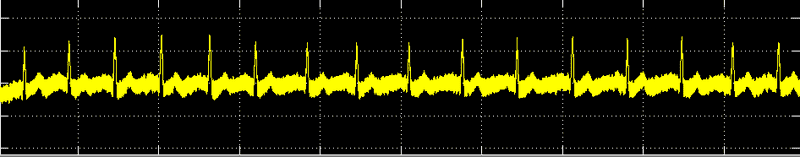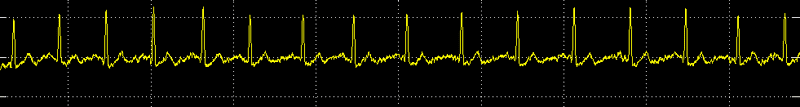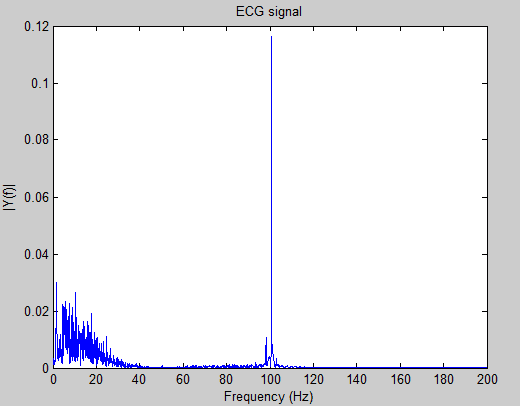Hi guys

i built an ECG system(preamplifier and filters), but i faced a problem in the filters:

the standard of ECG filters is 0.1 Hz cuttoff frequency for highpass filter and 100 Hz for lowpass filter

but i only obtain a good ECG signal when i make the cuttoff frequency of highpass filter 1 Hz and lowpass of cutoff frequency 80 Hz(8 order)

is my ECG filters correct(1 Hz and 80 Hz)?
or the filters cuttoff frequencies should be as the ECG filters standard?

i ask this question because i am writing a research paper.

When the cutoff frequencies were at 0.1 and 100 Hz, how is the waveform distorted? If you can post schematics and waveform captures we can better analyze the problem.

When the cutoff frequencies were at 0.1 and 100 Hz, how is the waveform distorted? If you can post schematics and waveform captures we can better analyze the problem.

ok ,this the ECG signal when the cutoff frequency of lowpass filter is 100 Hzand this the ECG signal when the cutoff frequency of lowpass filter is 80 HzLast edited:
The fact that the noise is reduced so dramatically by such a small shift in cutoff frequency is impressive and likely due to your use of an 8 order filter. First I would consider sources of 120 Hz such as the rectified power supply. Of course it may not be 120 Hz noise and it would help to expand the display to see if you can determine the frequency.

First I would consider sources of 120 Hz such as the rectified power supply. Of course it may not be 120 Hz noise and it would help to expand the display to see if you can determine the frequency.

i am not using power supply , i use two batteries (of 6 volt)

how can i determine the frequency noise?
and how can i prevent it from Interfere with ECG signal?

dlgoff
Gold Member
... how can i prevent it from Interfere with ECG signal?
Looks like you've done that. A schematic of the filters would be nice to see though.

Looks like you've done that. A schematic of the filters would be nice to see though.

i use digital filter(matlab) , my hardwere is only preamplifier.

Can you use Matlab to calculate the FFT of your waveform? That will give you a good idea what coefficients you should use for your filters.

Can you use Matlab to calculate the FFT of your waveform? That will give you a good idea what coefficients you should use for your filters.

ok dear i will do that as soon as, thank you

meBigGuy
Gold Member
I agree. The FFT will indicate what undesireable frequency components may be causing the noise. But, not where they are coming from.

The next questions will probably be: What is the sample rate of the system, and what are you using for an anti-alias filter?

For the FFT use as many points as you can and experiment with the windowing function for the best results.

NascentOxygen
Staff Emeritus
In a region where the mains frequency is 50Hz, it is very difficult to avoid picking up 50 Hz (and harmonics) on body electrodes even if your entire circuit is battery powered and has no reference to the mains.

Can you use Matlab to calculate the FFT of your waveform? That will give you a good idea what coefficients you should use for your filters.

I agree. The FFT will indicate what undesireable frequency components may be causing the noise.

this is the FFT of ECG signalit very clear that the noise 100 Hz com from power line harmonic

The next questions will probably be: What is the sample rate of the system, and what are you using for an anti-alias filter?
sample rate= 400

i am not using anti-alias filter,because the 100 Hz noise i can remove it in matlab

In a region where the mains frequency is 50Hz

yes the frequency of power line in my country is 50 Hz

it is very difficult to avoid picking up 50 Hz (and harmonics) on body electrodes even if your entire circuit is battery powered and has no reference to the mains.
No solutionWhy do you think the standard cutoff frequency is 100 Hz? Is the standard ECG designed for use in a 60 Hz country and the 100 Hz cutoff would then be effective for 120 Hz? I find it hard to believe that even when the heart is in fibrillation that you would have signals above 80 Hz. Can you think of any reason why an 80 Hz cutoff would not work?

berkeman
Mentor
How many leads are you using for the EKG pickups on the body? Can you post your schematic (as has been mentioned already). Do you have a ground lead as part of your circuit (like below). It's good that you are just battery-powering your circuit!

http://www.cs.wright.edu/~phe/EGR199/Lab_1/fig3.gif [Broken]

[Broken]

Last edited by a moderator:
berkeman
Mentor
Oh, and I agree that your upper cutoff frequency should ratio lower since the 100Hz number is likely meant for countries that use the 60Hz AC Mains frequency.

Why do you think the standard cutoff frequency is 100 Hz?
I read in many book, the ecg lowpass filter is 100 hz cutoff frequency

Why do you think the standard cutoff frequency is 100 Hz? Is the standard ECG designed for use in a 60 Hz country and the 100 Hz cutoff would then be effective for 120 Hz?
Yes, I think that

I find it hard to believe that even when the heart is in fibrillation that you would have signals above 80 Hz. Can you think of any reason why an 80 Hz cutoff would not work?
i also see that 80 Hz cutoff work properly but as i mentioned i am writing a research paper, my work must be
agree with a reference book or with another research paper

Last edited:
How many leads are you using for the EKG pickups on the body? Can you post your schematic (as has been mentioned already). Do you have a ground lead as part of your circuit (like below).

my schematic is like(similar) the schematic you put it:
http://www.cs.wright.edu/~phe/EGR199/Lab_1/fig3.gif [Broken]

Oh, and I agree that your upper cutoff frequency should ratio lower since the 100Hz number is likely meant for countries that use the 60Hz AC Mains frequency.

i agree with you tooLast edited by a moderator:
The power line waveform is thought of as being a sine wave, but what if there are harmonics present? What happens to those harmonics at your 400 Hz sampling rate?

Also consider what happens if you are sampling at an integer multiple of the power line frequency.